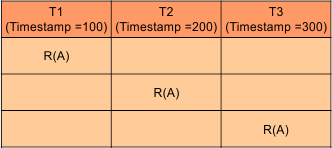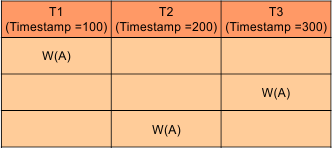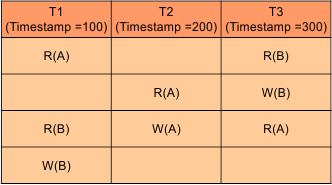Select Page

# Timestamp Ordering Protocol

• Timestamp is a some numeric value, when a transaction arrives in a schedule at any time then a timestamp value is assigned to it.
• The Timestamp Ordering Protocol is used to order the transactions based on their Timestamps.

Example: Suppose there are three transactions T1, T2, T3 and all are executing parallel on same data say (“A”) as

• T1 arrive at time 8:00oC So, assign as timestamp value as 100 called Older
• T2 arrive at time 8:10oC So, assign as timestamp value as 200 called Younger
• T3 arrive at time 8:15oC So, assign as timestamp value as 300 called Youngest

Keep in Mind, the transaction which came first will always execute first. We can also say the transaction which holds minimum timestamp value will execute first

Timestamp of any data is denoted by

TS (Ti)

Where TS is timestamp and Ti is transaction number.

Note: In rare cases the timestamp values may be given in descending orders as well. As youngest transaction in a schedule may holds the lowest timestamp value

## Timestamp Types

Timestamp of any data is  of two types

2. Write timestamp (WTS)

### 1. Read Timestamp of Data

Timestamp of last (latest) transaction number which performs the read operation on data (say “A”) successfully is called Read timestamp.

It is denoted by RTS (“data”) = timestamp-Value.

Example:As in above diagram T3 is the latest transaction which perform the read operation. So Read timestamp of data “A” will be the Read timestamp of least transaction.

RTS (“A”) = 300

### 2. Write Timestamp of Data

Timestamp of last (latest) transaction number which performs the write operation on same data successfully is called Write timestamp.

It is denoted by WTS (Ti) = timestamp-Value.

Example:As in above diagram T2 is the latest transaction which perform the Write operation on same data “A”. So write timestamp of some data (Say “A)” will be.

WTS (A) = 200

If there are multiple data items in the schedule (i.e. A, B, C…) then each data item holds its own Read and Write timestamp as given below• RTS(A) = 300
• WTS(A) =200
• RTS(B) =100
• WTS(B) =100

## Timestamp Ordering Protocol Rules

Timestamp follow some rules to perform read or write operation. The rules are also known as Thomas rules.

Rule No 01 is used when any transaction wants to perform Read(A) operation

• If WTS(A) > TS (Ti), then Ti Rollback
• Else (otherwise) execute R(A) operation and SET RTS (A) = MAX {RTS(A), TS(Ti)}

Rules No.2 is rules is used when a transaction needs to perform WRITE (A)

• If RTS(A) > TS (Ti), then Ti Rollback.
• If WTS(A) > TS (Ti), then Ti Rollback.
• Else (otherwise) execute W(A) operation and SET WTS (A) = TS(Ti).

Where “A” is some data

Timestamp protocol always ensure Serilizabilty and Deadlock removal because Transaction with smaller timestamp (TS) will came first in execution sequence than Transaction with higher TS.

• If T1 timestamp = 100
• T2 timestamp = 200
• T3 timestamp = 300

Then transaction execution sequence is given below

• Schedule may not be recoverable.
• Schedule may not be cascading free.

Help Other’s By Sharing…